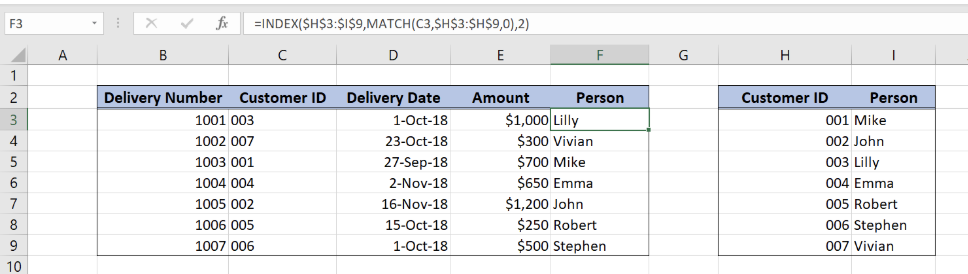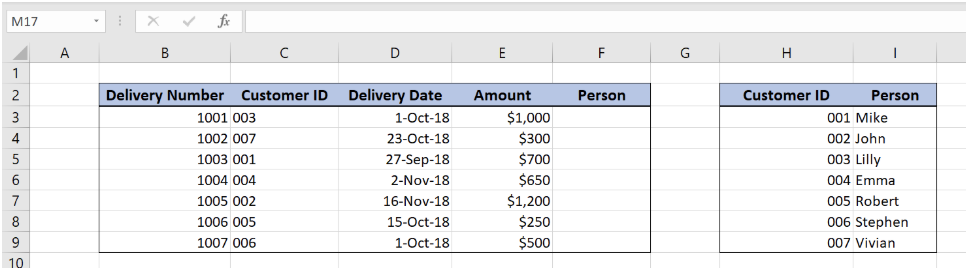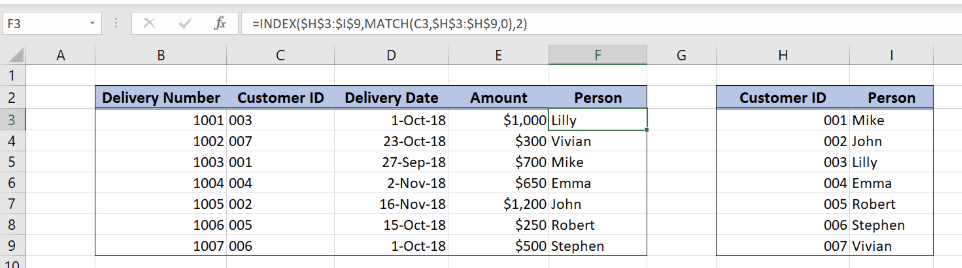Get instant live expert help with Excel or Google Sheets“My Excelchat expert helped me in less than 20 minutes, saving me what would have been 5 hours of work!”

#### Post your problem and you’ll get Expert help in seconds.

Your message must be at least 40 characters
Our professional Expert are available now. Your privacy is guaranteed.

# How to Join Tables in Excel with INDEX and MATCH

Excel allows us to join tables with INDEX and MATCH functions. The MATCH function returns a row value in a table, while the INDEX returns a value for that row. This step by step tutorial will assist all levels of Excel users in joining tables using INDEX and MATCH functions.Figure 1. The final result of joining tables with INDEX and MATCH functions

## Syntax of the INDEX formula

`=INDEX(array, row_num, column_num)`

The parameters of the INDEX function are:

• array – a range of cells where we want to get a data
• row_num – a number of a row in the array for which we want to get a value
• column_num – a column in the array which returns a value.

## Syntax of the MATCH formula

`=MATCH(lookup_value, lookup_array, [match_type])`

The parameters of the MATCH function are:

• lookup_value – a value which we want to find in the lookup_array
• lookup_array – the array where we want to find a value
• [match_type] – a type of match. We put 0 which is an exact match.

## Setting up Our Data for Joining the Tables with INDEX and MATCH

Our table consists of 5 columns: “Delivery Number” (column B), “Customer ID (column C), “Delivery Date” (column D), “Amount” (column D) and “Person” (column F).

In column F, we want to populate a Person from the Lookup table in the range H3:I9. This table consists of 2 columns: “Customer ID” (column H) and “Person” (column I). Two tables are joined by the columns “Customer ID”.Figure 2. Data that we will use in the joining tables example

## Join two tables using INDEX and MATCH

We want to get a person in the cell F3, from the lookup table H3:I9, based on the Customer ID 003 in the cell C3.

The formula looks like:

`=INDEX(\$H\$3:\$I\$9, MATCH(C3, \$H\$3:\$H\$9, 0), 2)`

The array is the range H3:I9. The row_num is the result of the `MATCH(C3, \$H\$3:\$H\$9, 0)`, which returns the row of 003 Customer ID in the lookup range.

To apply the SUMIFS function, we need to follow these steps:

• Select cell F3 and click on it
• Insert the formula: `=INDEX(\$H\$3:\$I\$9, MATCH(C3, \$H\$3:\$H\$9, 0), 2)`
• Press enter
• Drag the formula down to the other cells in the column by clicking and dragging the little “+” icon at the bottom-right of the cell.Figure 3. Using the INDEX and MATCH functions to join two tables

The MATCH function will return 3, as Customer ID 003 is in the third row of the lookup range. This is the row_num parameter of the INDEX function. The final result in the cell F3 is Lily as this is the value of the Person column in the third row.

Most of the time, the problem you will need to solve will be more complex than a simple application of a formula or function. If you want to save hours of research and frustration, try our live Excelchat service! Our Excel Experts are available 24/7 to answer any Excel question you may have. We guarantee a connection within 30 seconds and a customized solution within 20 minutes.

### Did this post not answer your question? Get a solution from connecting with the expert.Another blog reader asked this question today on Excelchat:
Solution examplesindex and match with duplicates. I need to use another column as a reference, so my return value has two match the value of two things for it to return
Solved by E. H. in 60 minsI need a formula that will check if 2 or more cells containing names match then add the adjacent cells to matching name together.
Solved by F. D. in 59 minsHello, I have a big spreadsheet in which I need to know how many patients came in each month based on provider. I am using the following formula but I still get the N/A error. =INDEX('No Show Appts Data'!D:E, MATCH(1, 'No Show Appts Data'!D:D='No Shows Data'!M2)*('No Shows Data'!E:E='No Shows Data'!N1),0)) No Show Appts Data is the name of the sheet where Column D is Month Year of date in question and Column E is the provider. No Shows Data is the sheet where I am making all the formula calculation where Column M is Month and Year and Column N is the provider in question and therefore M2 is the month in question and N1 the provider in question. How do I fix this error? Thanks
Solved by F. H. in 40 minsI need to find an INDEX function that will convert the Call Day (a number 1-7) to the actual weekday found in row 1 of the DayofWeek named range. I don't know if I'm supposed to use the MATCH function as well or not.
Solved by D. D. in 11 minsI have two columns with names and a third one with email addresses. For every name that matches, I need to copy the email address to an empty column next to the matching name. .
Solved by E. U. in 15 mins## Subscribe to Excelchat.coAnother blog reader asked this question today on Excelchat: